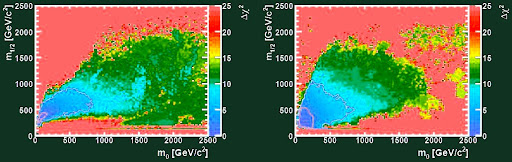## Tuesday, August 25, 2009

### SUSY masses near 120 GeV favored

First, congratulations to the 400th birthday of Galileo Galilei's astronomical telescope!

In July 2009, Buchmueller et al., a team of 10 theorists and experimenters including John Ellis, published an interesting frequentist analysis of the likely masses of new particles in supersymmetric models of particle physics:
Likelihood functions for supersymmetric observables in frequentist analyses of the CMSSM and NUHM1 (click)
They focus on two models, constrained MSSM (CMSSM) and a non-universal Higgs mass model (NUHM1). Roughly speaking, they're two lower-dimensional slices in the MSSM parameter space, assuming that the spin-0 and lightest spin-1/2 superpartners have masses easily calculated from two parameters. Such artificially more specific submodels are useful to get an idea what's predicted by the MSSM.

The authors have used a frequentist analysis to estimate the probability distribution. It means that they calculate a "score" as a function of the parameter spaces. The lower score, the better (more likely) for a point in the parameter space. The "score" is schematically
chi-square = sum [(experiment-theory)/error]^2
Note that in general, "theory" is a complicated function of the parameters predicted for the quantity by the theory. The quantity is measured in an "experiment", with an "error" margin. Additional terms from other considerations may be added to this "score".

The probabilistic distribution may be obtained as a chi-square distribution from the calculated function chi-square, as a function of the parameters, taking the right number of parameters into account (it affects the power law which multiples exp(-chi^2/2), the basic factor generalizing the normal distribution).Click to zoom in.

When this process is completed for many high-precision quantities, they may offer some predictions for the masses of the new particles. For example, the universal (by assumption) masses of spin-0 and spin-1/2 are visualized on the graph above. The left chart refers to CMSSM while the right chart depicts NUHM1. You see that the masses are likely to be below 600 GeV or so, and the spin-0 masses in CMSSM are likely to be below 150 GeV.

Both the Higgs boson and a neutralino is favored near 120 GeV (and one of the models prefers such values even if you ignore the LEP constraints). The best stau mass estimates are close to 130 GeV. Tan(beta) is preferred around 10. The branching ratio of B_s to a muon pair is strongly favored near 4 parts per billion. However, gluinos are between 500 and 1,500 GeV, about 0-150 GeV heavier than the five light squark flavors.

Many other figures - and correlations between various pairs of unknown masses - are discussed in the paper, too. The announced numbers generally imply that if low-energy SUSY is there, it is more likely than not that the LHC will discover it pretty soon - literally within weeks after the collider is re-activated in November 2009.

Hat tip: Tommaso Dorigo, a supersymmetry foe who is gradually turning his coat, too ;-)